# (1 point) Suppose that the matrix A has repeated eigenvalue with the following eigenvector and generalized...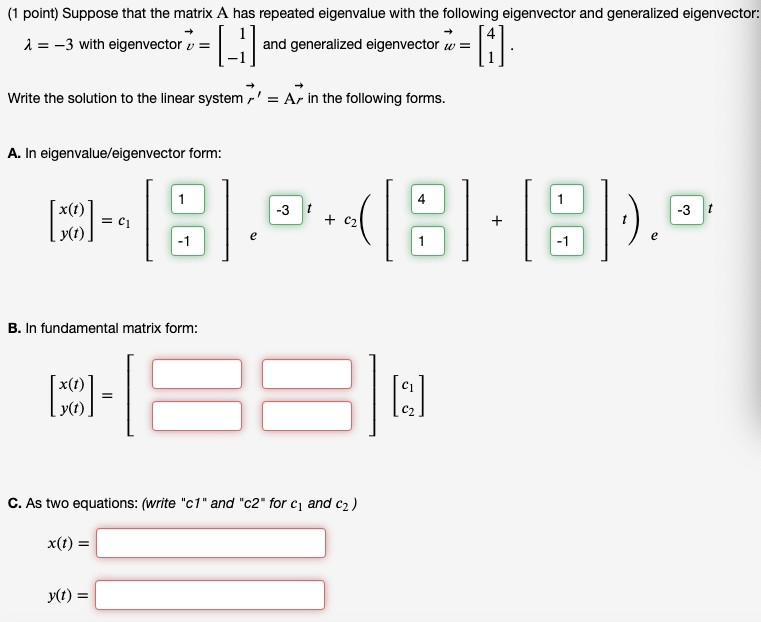(1 point) Suppose that the matrix A has repeated eigenvalue with the following eigenvector and generalized eigenvector: i = -3 with eigenvector v = and generalized eigenvector w= = [-] =  Write the solution to the linear system r' = Ar in the following forms. A. In eigenvalue/eigenvector form: 1 (O) = - 18.05.8:8)... y(t) B. In fundamental matrix form: (O)- x(1) y(t) [:] C. As two equations: (write "c1" and "c2" for c and c2) X(t) = yt) =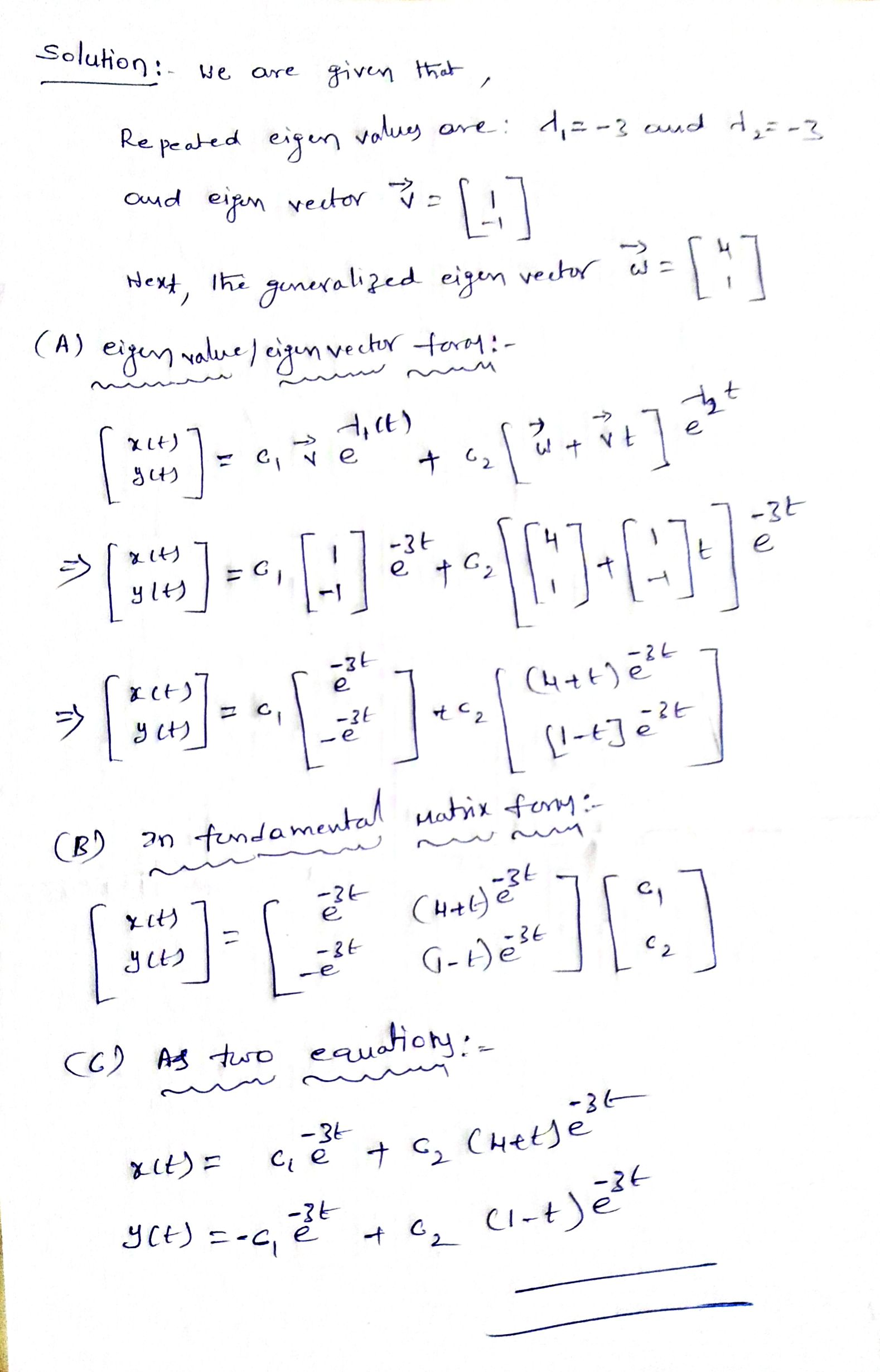##### Add Answer of: (1 point) Suppose that the matrix A has repeated eigenvalue with the following eigenvector and generalized...
Similar Homework Help Questions
• ### (1 point) Suppose that the matrix A has repeated eigenvalue with the following eigenvector and generalized...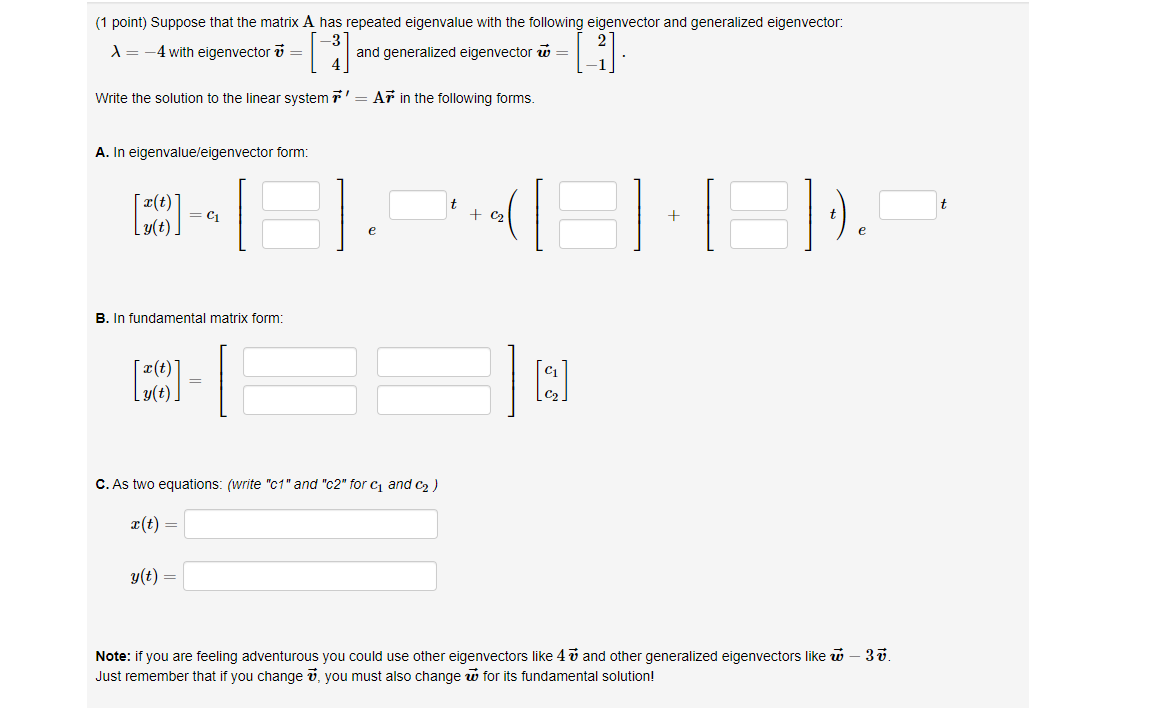(1 point) Suppose that the matrix A has repeated eigenvalue with the following eigenvector and generalized eigenvector: X= -4 with eigenvector v = and generalized eigenvector ū= [] (-1) Write the solution to the linear system r' = Ar in the following forms. A. In eigenvalue/eigenvector form: t t [CO] = C1 + C2 + I g(t). e . - 1 B. In fundamental matrix form: [CO] C. As two equations: (write "c1" and "c2" for 1 and 2) X(t)...

• ### (1 point) Suppose that the matrix A has the following eigenvalues and eigenvectors: 4 = 2...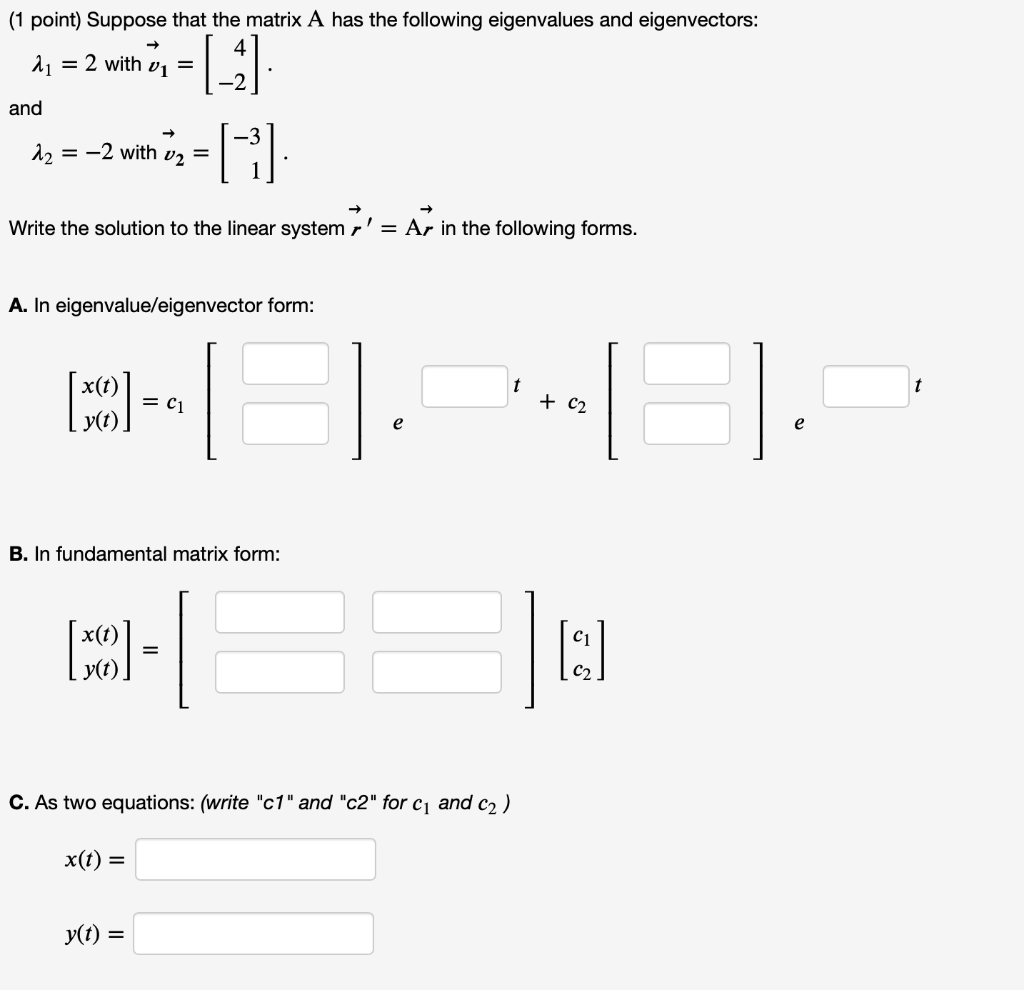(1 point) Suppose that the matrix A has the following eigenvalues and eigenvectors: 4 = 2 with vi = and |_ G 12 = -2 with v2 = Write the solution to the linear system r' = Ar in the following forms. A. In eigenvalue/eigenvector form: x(t) (50) = C1 + C2 e e B. In fundamental matrix form: (MCO) = I: C. As two equations: (write "c1" and "c2" for C1 and c2) x(t) = yt) =

• ### Suppose that the matrix A A has the following eigenvalues and eigenvectors: (1 point) Suppose that...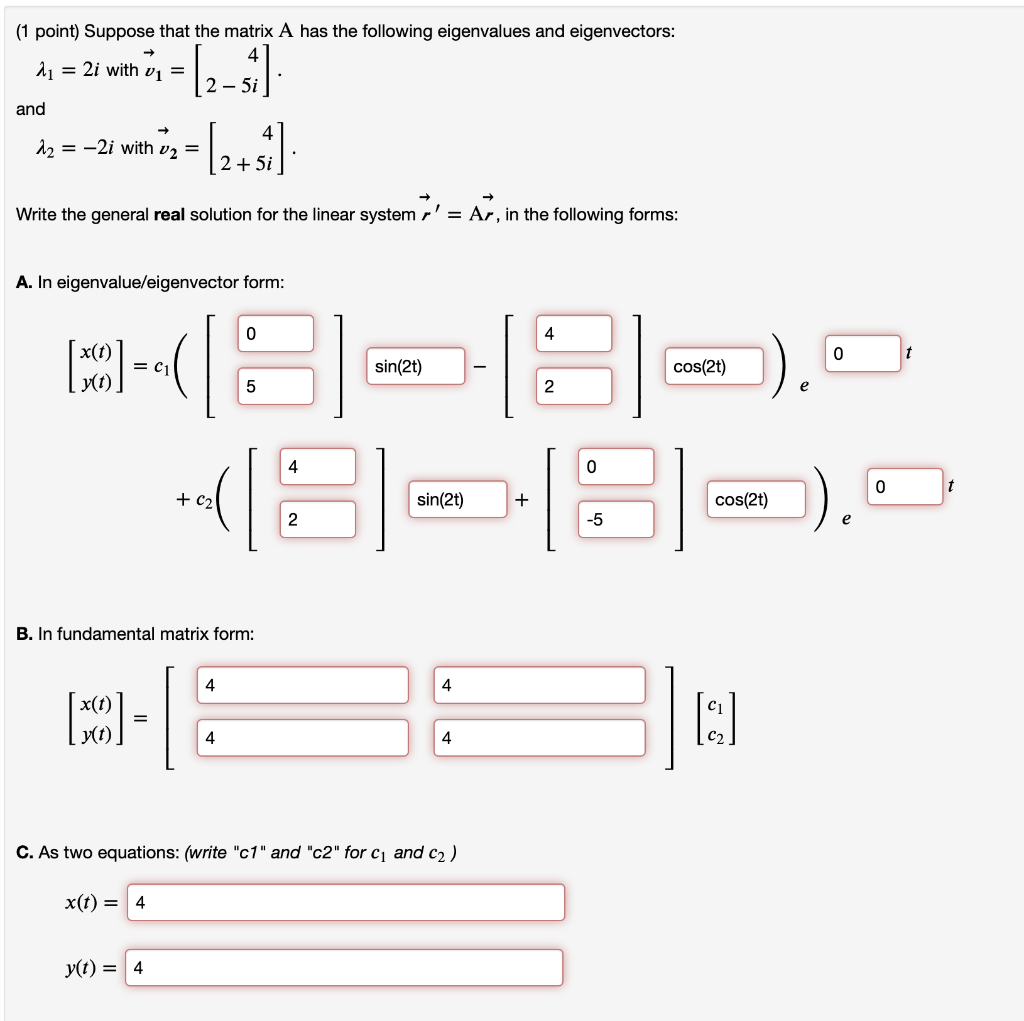Suppose that the matrix A A has the following eigenvalues and eigenvectors: (1 point) Suppose that the matrix A has the following eigenvalues and eigenvectors: 2 = 2i with v1 = 2 - 5i and - 12 = -2i with v2 = (2+1) 2 + 5i Write the general real solution for the linear system r' = Ar, in the following forms: A. In eigenvalue/eigenvector form: 0 4 0 t MODE = C1 sin(2t) cos(2) 5 2 4 0 0...

• ### (1 point) Suppose that the matrix A has the following eigenvalues and eigenvectors: A1 = 4...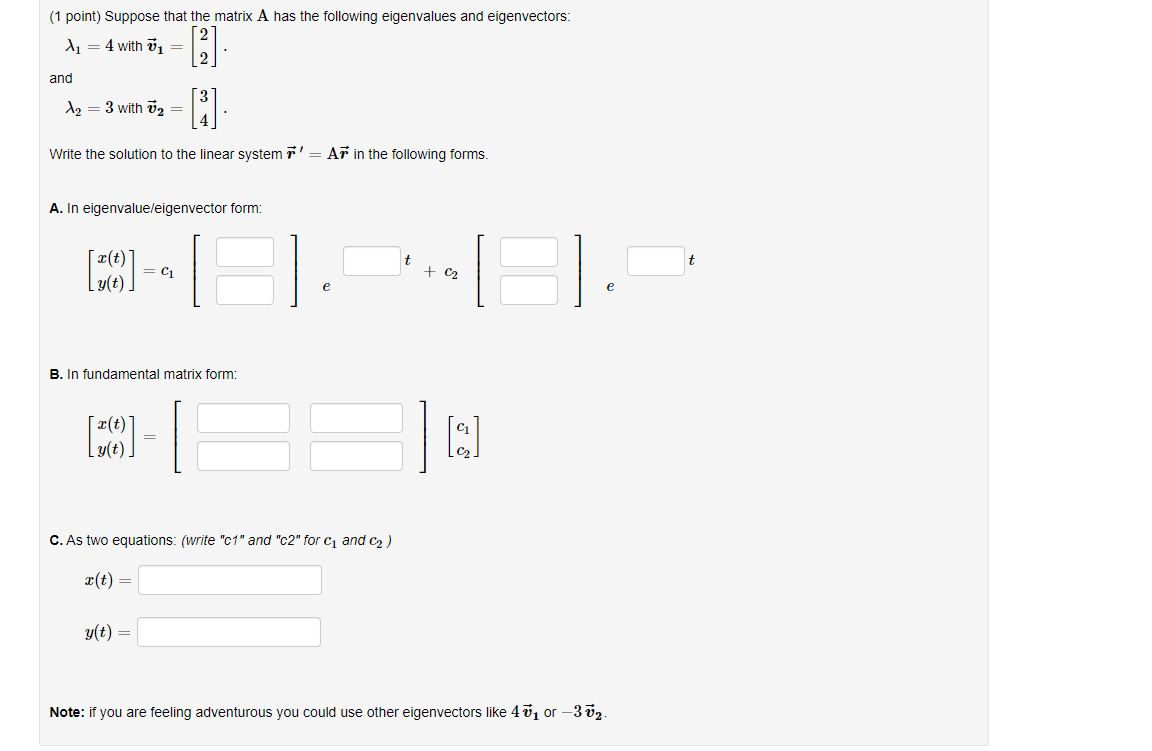(1 point) Suppose that the matrix A has the following eigenvalues and eigenvectors: A1 = 4 with = and  [i] Az = 3 with Ū2 = Write the solution to the linear system r' = Ar in the following forms. A. In eigenvalue/eigenvector form: t (10) -- + C2 e e B. In fundamental matrix form: (39) - g(t). C. As two equations: (write "c1" and "c2" for C and C2) X(t) = g(t) = Note: if you are...

• ### (1 point) Suppose that the matrix A has the following eigenvalues and eigenvectors 2-2i and -2+2i Write the solution to...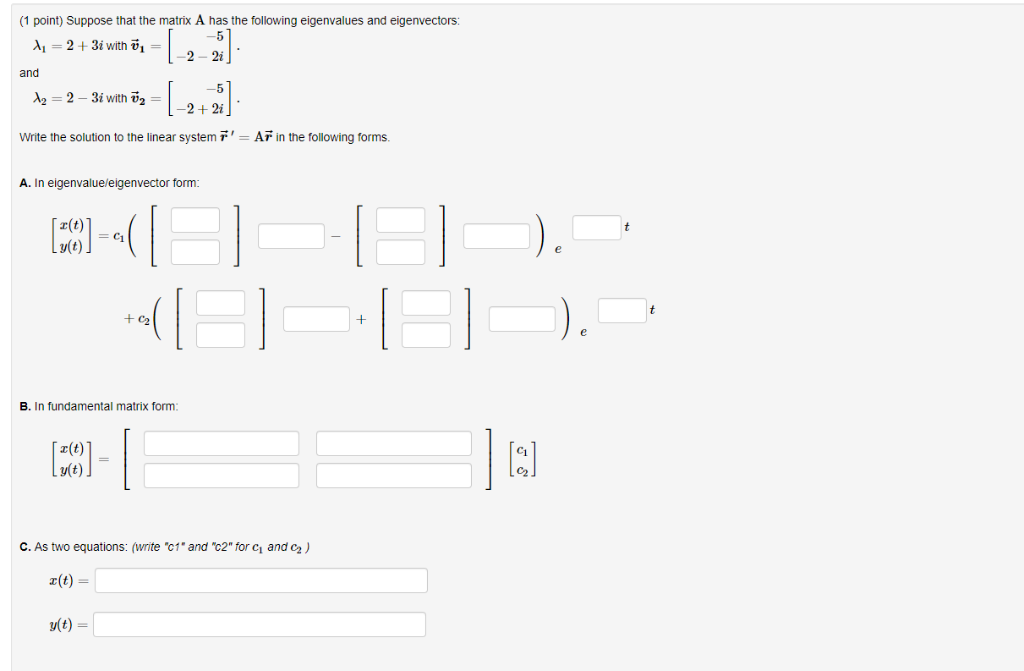(1 point) Suppose that the matrix A has the following eigenvalues and eigenvectors 2-2i and -2+2i Write the solution to the linear system AF in the following forms A. In eigenvalueleigenvector form r(t) B. In fundamental matrix form z(t) v(t) C. As two equations: (write "c1* and "c2" for ci and C2) a(t)- v(t)- (1 point) Suppose that the matrix A has the following eigenvalues and eigenvectors 2-2i and -2+2i Write the solution to the linear system AF in the...

• ### (1 point) Consider the initial value problem -51เซี. -4 มี(0) 0 -5 a Find the eigenvalue λ, an eigenvector ul and a gen...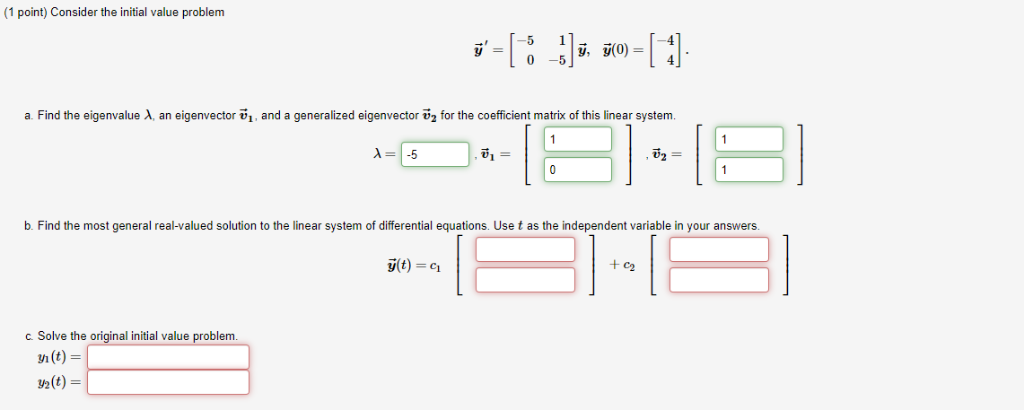(1 point) Consider the initial value problem -51เซี. -4 มี(0) 0 -5 a Find the eigenvalue λ, an eigenvector ul and a generalized eigenvector u2 for the coefficient matrix of this linear system -5 u2 = b. Find the most general real-valued solution to the linear system of differential equations. Use t as the independent variable in your answers c2 c. Solve the original initial value problem m(t) = 2(t)- (1 point) Consider the initial value problem -51เซี. -4 มี(0)...

• ### (1 point) Consider the initial value problem 7=[8_5]: x0=(-3) Find the eigenvalue 1, an eigenvector vi,...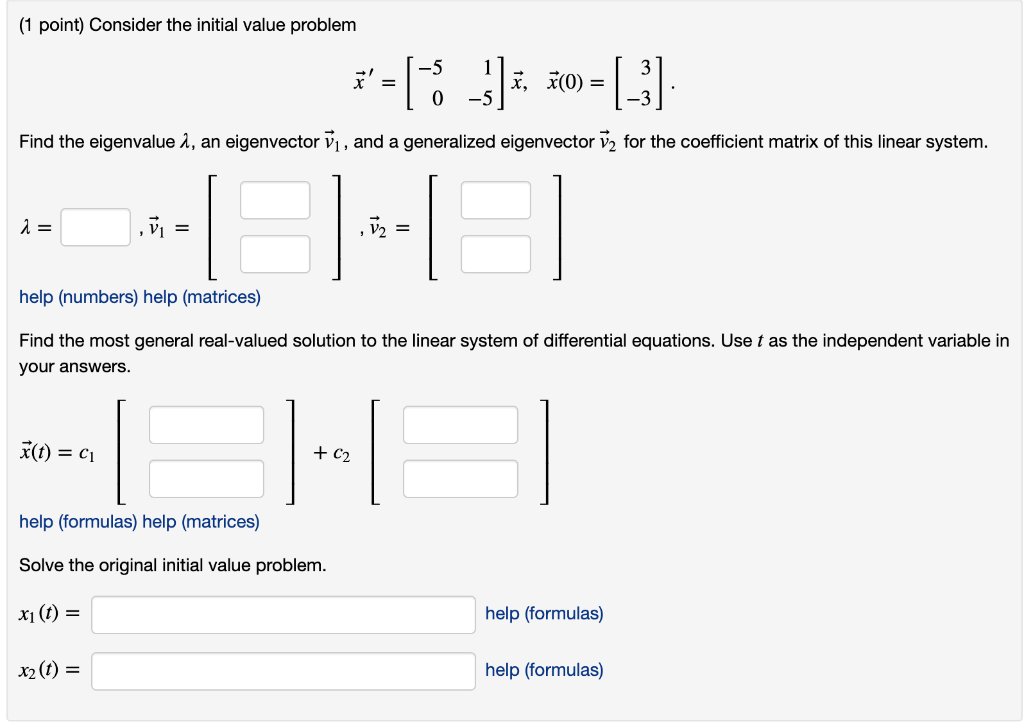(1 point) Consider the initial value problem 7=[8_5]: x0=(-3) Find the eigenvalue 1, an eigenvector vi, and a generalized eigenvector v2 for the coefficient matrix of this linear system. a = vi = help (numbers) help (matrices) Find the most general real-valued solution to the linear system of differential equations. Use t as the independent variable in your answers. F(t) = 61 IHO + C2 help (formulas) help (matrices) Solve the original initial value problem. xu(t) = help (formulas) x2...

• ### (1 point) Consider the initial value problem -2 j' = [ y, y(0) +3] 0 -2...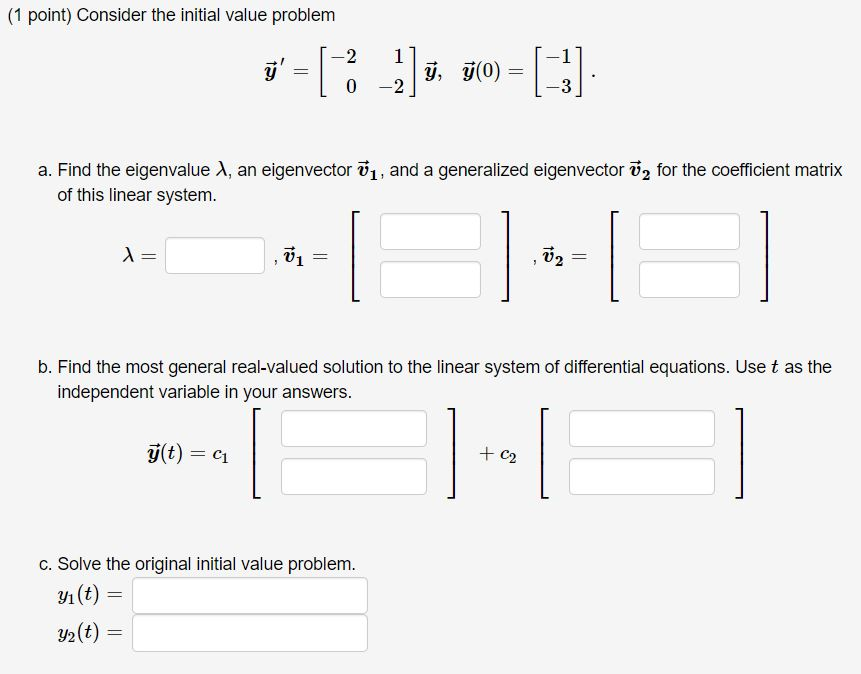(1 point) Consider the initial value problem -2 j' = [ y, y(0) +3] 0 -2 a. Find the eigenvalue 1, an eigenvector 1, and a generalized eigenvector ū2 for the coefficient matrix of this linear system. = --1 V2 = b. Find the most general real-valued solution to the linear system of differential equations. Use t as the independent variable in your answers. g(t) = C1 + C2 c. Solve the original initial value problem. yı(t) = y2(t) ==

• ### مل 3 (1 point) Suppose that a 2 x 2 matrix A has an eigenvalue 3...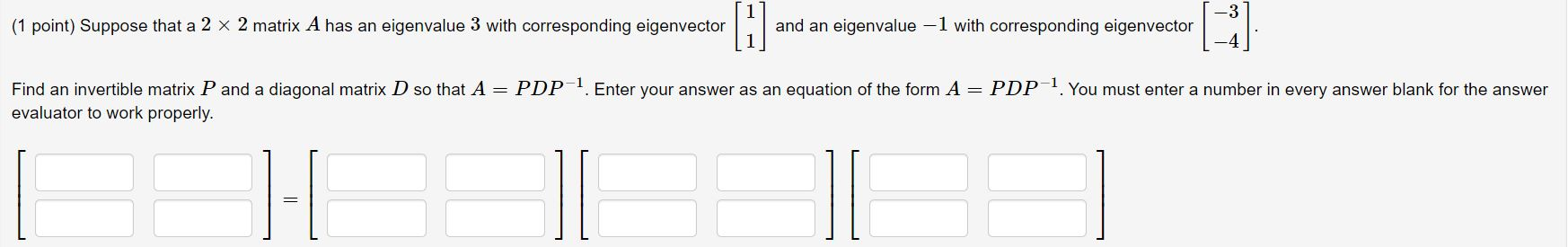مل 3 (1 point) Suppose that a 2 x 2 matrix A has an eigenvalue 3 with corresponding eigenvector and an eigenvalue -1 with corresponding eigenvector Find an invertible matrix P and a diagonal matrix D so that A = PDP-1. Enter your answer as an equation of the form A = PDP-1. You must enter a number in every answer blank for the answer evaluator to work properly. 1-1

• ### 2. (10 points) Suppose v is an eigenvector of A with eigenvalue X, and let c...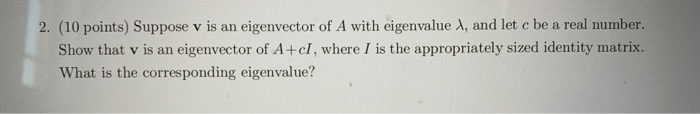2. (10 points) Suppose v is an eigenvector of A with eigenvalue X, and let c be a real number. Show that v is an eigenvector of A+cI, where I is the appropriately sized identity matrix. What is the corresponding eigenvalue?

Free Homework App